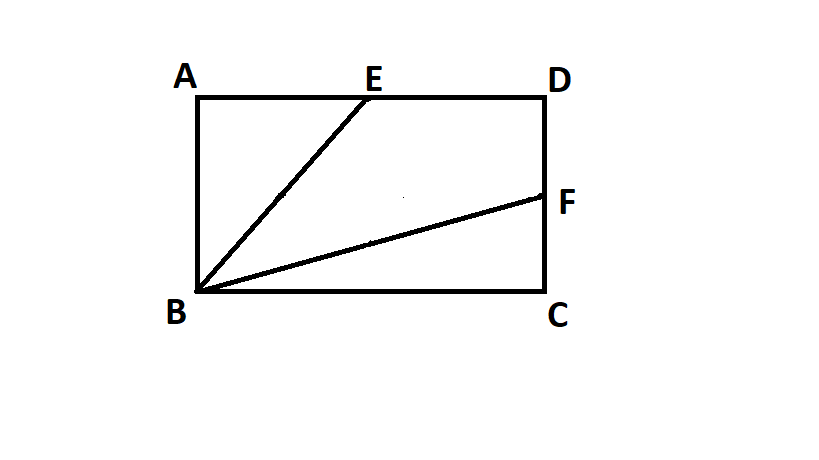# Can you find the area?

Geometry Level 1HEY!! suppose that,ABCD is a rectangle.E,F are the midpoints of side AD and CD respectively.If the AREA of quadrilateral EBFD is 9.What is the area of the rectangle ABCD?

×